Courses
Courses for Kids
Free study material
Free LIVE classes
More

# Square Unit: A Metric Unit Used to Measure Area## What is a Square Unit?

A square unit is defined as a metric unit used to measure area.

For Example: Square Metres (m2), Square Centimetres (cm2), Square Inches (In2), Square Feet (ft2), et.c are all square measures.

The dog below is patiently sitting inside a one square metre area.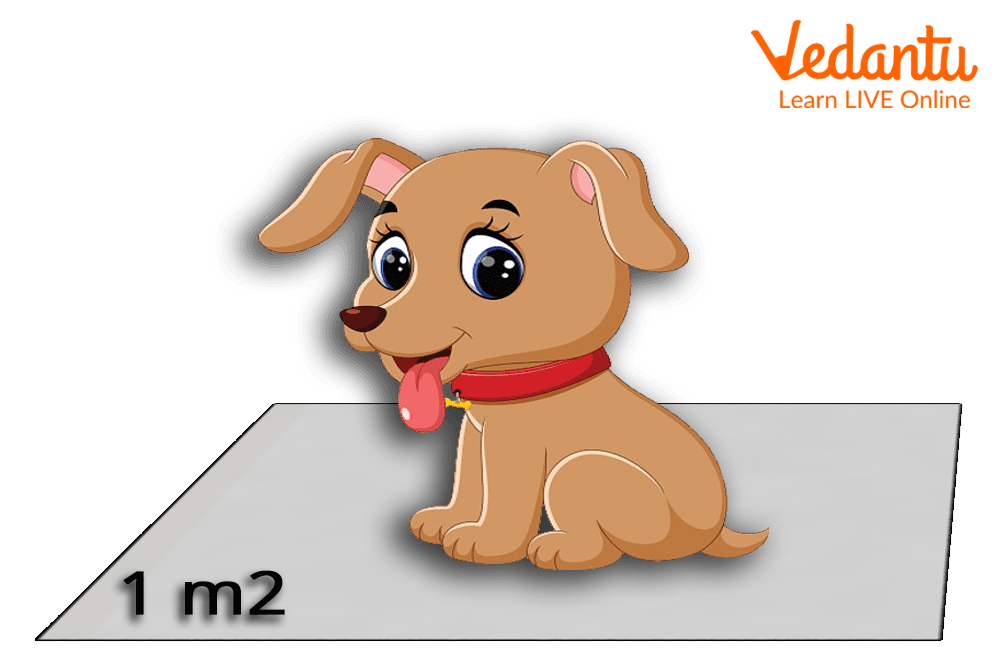Image: A dog sitting in one square metre

## What is One Square Unit?

One square unit or a square unit is equal to the area of a square having a side length of 1 unit. The figure below represents the square that has an area of one square unit.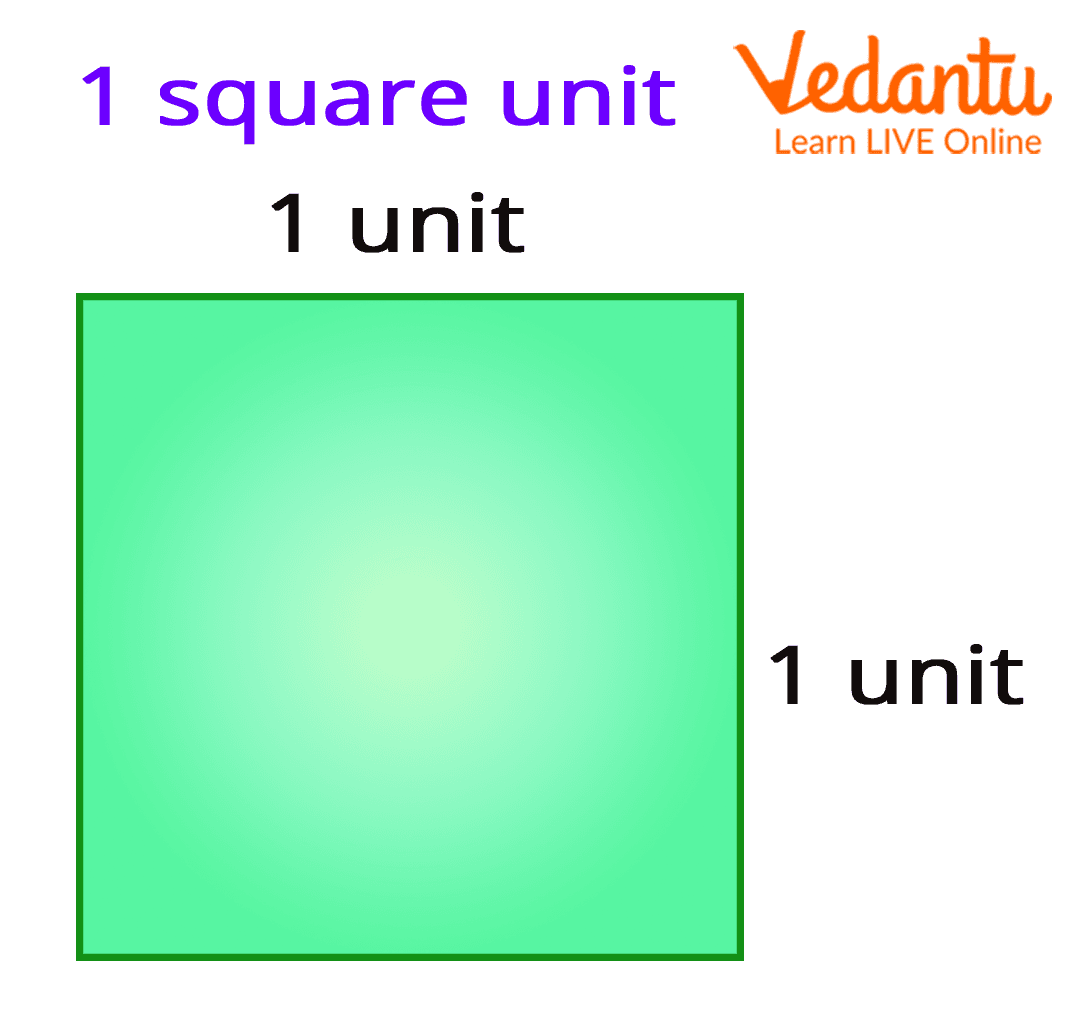Image: A square with side 1 unit

Note: The square unit can also be written as (unit)2 or sq unit.

## Measuring Area Using Square Unit

The area is defined as the number of square units that covers the surface of a closed figure. Area is always measured in square units such as square metre, square centimetres, etc.

Example: What is the area of the following shape?

Just count the number of squares used to cover the surface of a shape. That’s the area.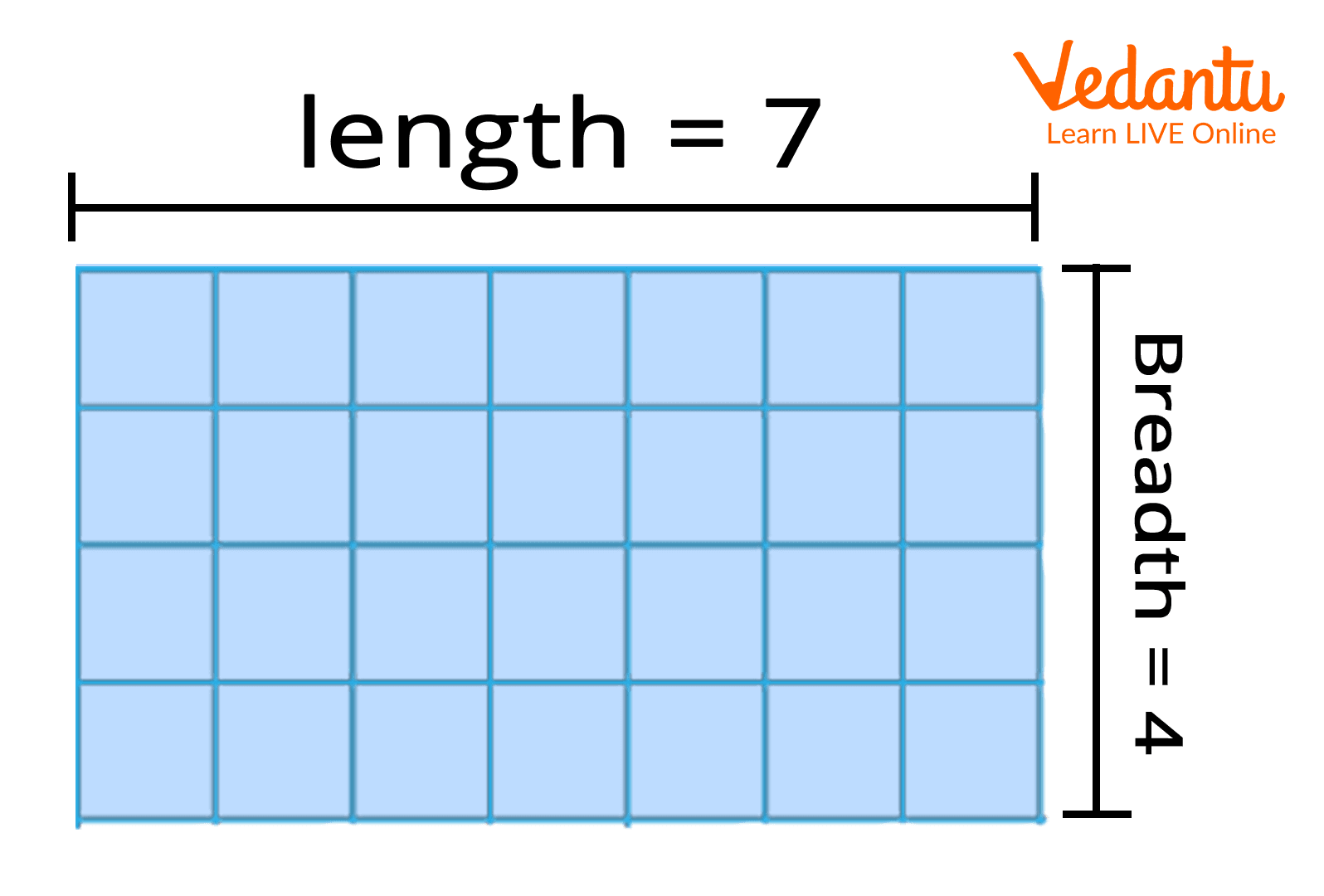Image: Area of Rectangle

So, what’s the area of the above rectangle?

It is 28 square units.

There is another way to find an area.

Just multiply the length and breadth of a rectangle.

The length is 7 and the breadth is 4.

= 7 4

= 28 square units

Therefore, the area of the rectangle is 28 square units.

## How Big is Square Units?

Suppose you have a playing room with an area of 9 square units? And, you need to cover the entire floor of the playing room with a rubber mat. Knowing the number of square units will not help you because you don’t know how big each square unit is? For this, you need to know the unit of area. The unit of area will actually tell you how much space the shape actually covers.

Remember, there are different units of areas. We use square centimetres and square inches for measuring small surfaces like pictures, drawing, photo frames, etc.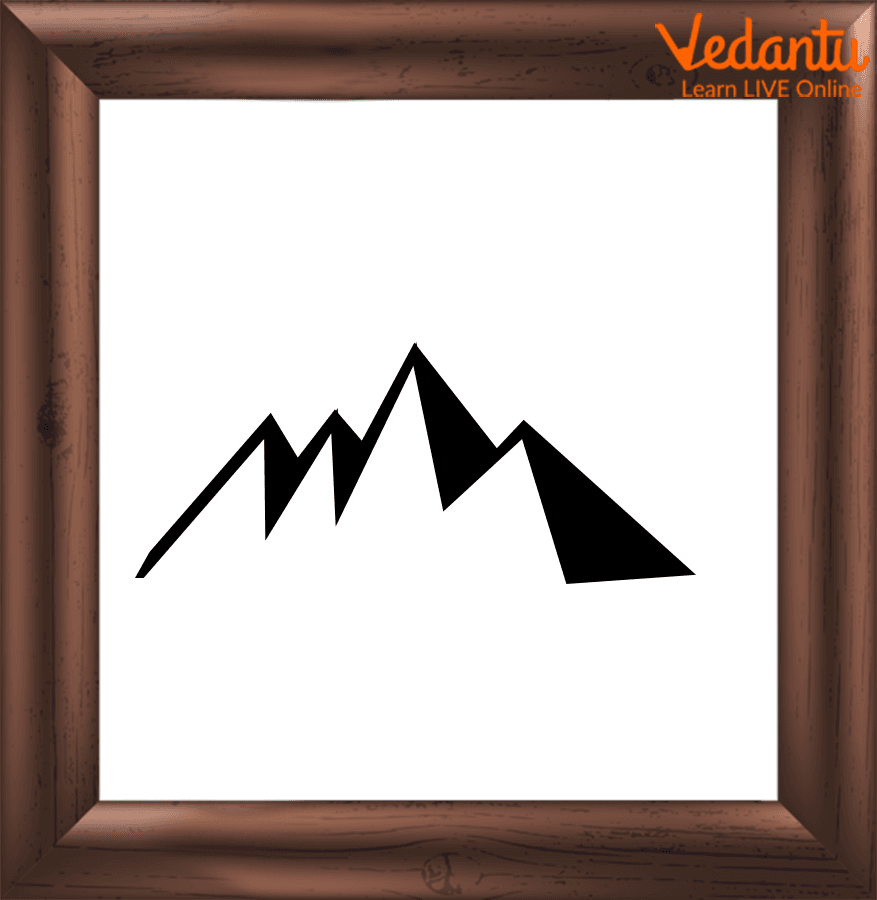Image: A square shaped Photo frame

We use square millimetres for measuring very tiny objects like fingernails.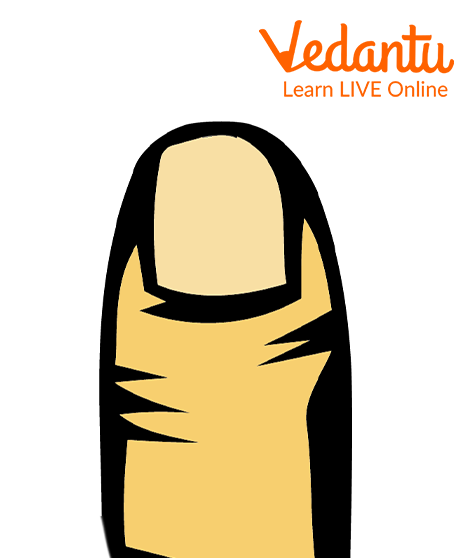Image: A fingernail

We use square feet, square yard, and square metres for measuring big spaces like the area of the garden, house, fields, etc.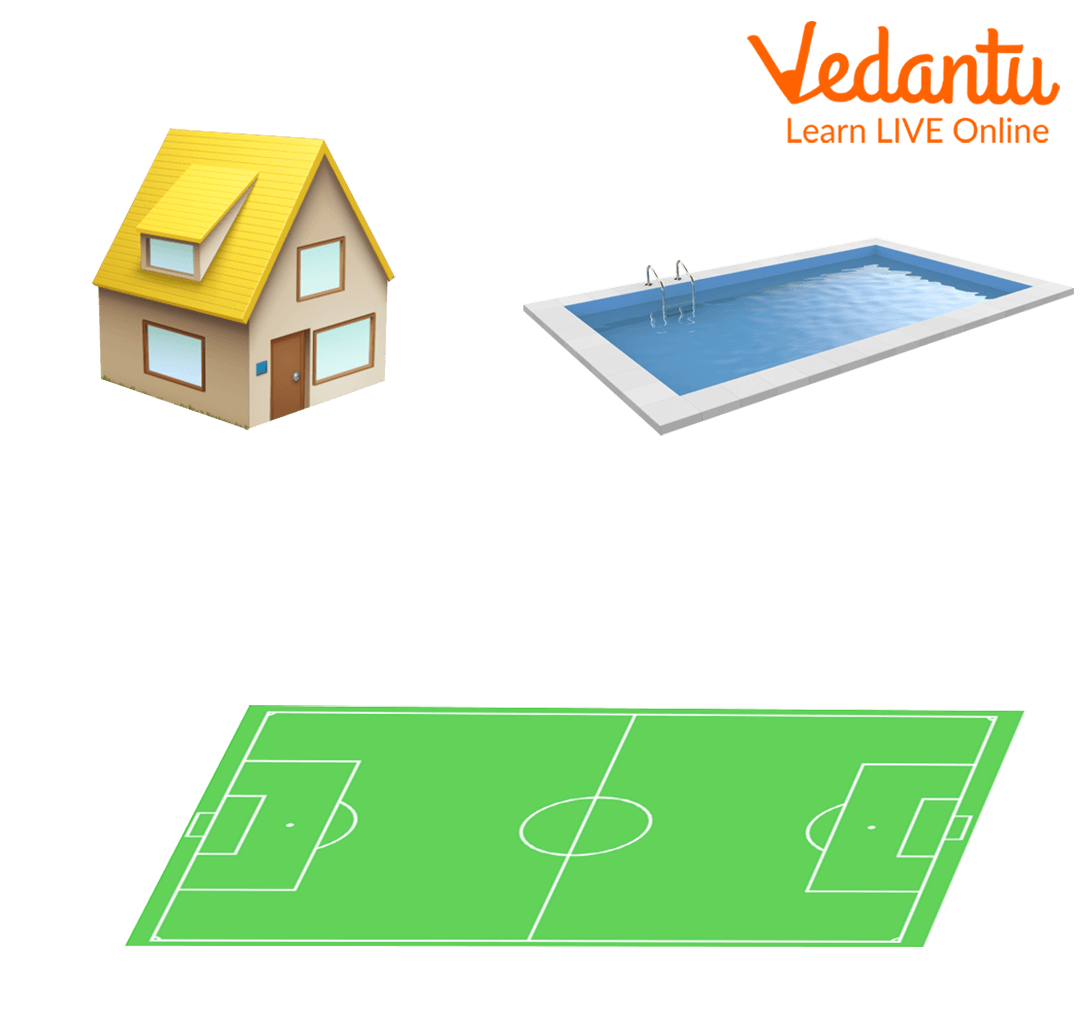Image: Area of big spaces

We use square kilometres and square miles for measuring large areas of land. Sometimes hectares and acres are also used to measure the area of land.

## Shorter Ways of Writing Square Units

Following are the abbreviations of different square units:

• Square Feet (Ft2)

• Square Kilometres (Km2)

• Square Inches (In2)

• Square Centimetres (Cm2)

• Square Millimetres (Mm2)

• Square Metres (M2)

• Square Yards (Yd2)

• Square Miles (Mi2)

## Customary and Metric Square Units

Let us look at the different customary and metric square units.

### Customary Square Units

The customary square units are used in the United States. The customary square units are:

• Square Inches (In2)

• Square Miles (Mi2)

• Square Yards (Yd2)

• Square Feet (Ft2)

### Metric Square Units

The metric square units are used in most of the countries throughout the world. The metric square units are:

• Square Millimetres (Mm2)

• Square Centimetres (Cm2)

• Square Metres (M2)

• Square Kilometres (Km2)

## Did You Know?

Square units are often misinterpreted with unit squares. The square unit is a unit of measurement, whereas a unit square is a square with side measuring 1 unit.

## Conclusion

In this article, we have learnt square units, different types of square units, how big is square unit, and many more. We hope that this article helps children better understand the term square unit.

Last updated date: 18th Sep 2023
Total views: 135.6k
Views today: 4.35k

## FAQs on Square Unit: A Metric Unit Used to Measure Area

1. Why is the area expressed in square units?

Area is expressed and measured in square units. This is because the area of geometrical shape is the number of square units required to completely cover it like square tiles on a floor. Area of square is calculated using the square formula side times side. As each side of the square is the same, it can be simply calculated by squaring the length of one side.

2. What is the relationship between area and square units?

In Geometry, the area of any two-dimensional object is the amount of space occupied by the object. The standard unit used to measure area is square unit and is generally represented in square metre, square centimetre, square inches, square feet, etc. On the other hand, a square unit is a square with a side of length one unit.